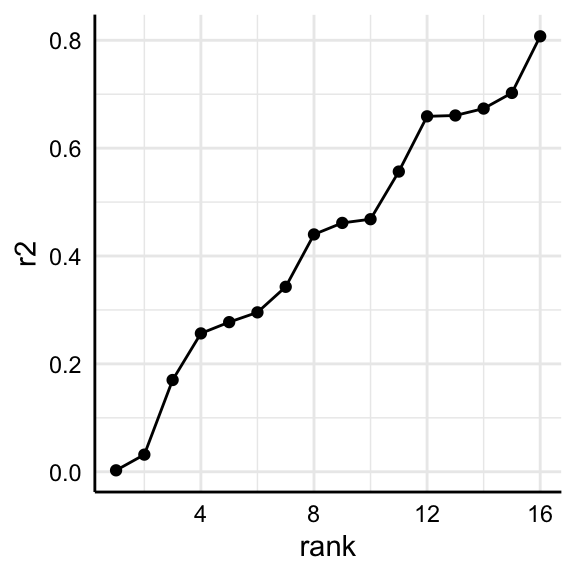Investigating selected specifications

Per default, run_specs() summarizes individual specifications by using broom::tidy(). For most cases, this provides “good-enough” summaries of the relationship of interest. Sometimes, however, it might be useful to investigate specific models in more detail or to investigate a specific parameter that is not provided by broom::tidy() (e.g., r-square, AIC). This vignette shows how to access individual models.

1. Run specification curve analysis

If we want to investigate individual models and particularly all aspects of that model, we need to use run_specs() with the additional argument keep.results = TRUE.

library(specr)
library(dplyr)
library(purrr)
library(ggplot2)
library(performance)

# Run specification curve analysis
results <- run_specs(df = example_data,
y = c("y1", "y2"),
x = c("x1", "x2"),
model = c("lm"),
controls = c("c1", "c2"),
subsets = list(group1 = unique(example_data$group1), group2 = unique(example_data$group2)),
keep.results = TRUE)

2. Identify model(s) of interest

For this example, we are going to look at two specific models (same independent variables, all controls, all participants, but different dependent variables).

(y_models <- results %>%
filter(x == "x1",
controls == "c1 + c2",
subsets == "all"))
#> # A tibble: 2 x 13
#>   x     y     model controls res   estimate std.error statistic  p.value
#>   <chr> <chr> <chr> <chr>    <lis>    <dbl>     <dbl>     <dbl>    <dbl>
#> 1 x1    y1    lm    c1 + c2  <lm>    6.17       0.412    15.0   4.70e-42
#> 2 x1    y2    lm    c1 + c2  <lm>   -0.0545     0.267    -0.204 8.38e- 1
#> # … with 4 more variables: conf.low <dbl>, conf.high <dbl>, obs <int>,
#> #   subsets <chr>

As you can see, the resulting tibble includes an additional column called “res”. This column includes the entire “model object” and we can use it to further investigate each model.

3. Investigate models

For example, we can now easily get a full summary of the models and compare individual coefficients and statistics.

y_models %>%
pull(res) %>%
map(summary) %>%
map(coef)
#> []
#>               Estimate Std. Error   t value     Pr(>|t|)
#> (Intercept) -13.155786  1.2877892 -10.21579 2.305561e-22
#> x1            6.167539  0.4121184  14.96545 4.697951e-42
#> c1            3.305519  0.2120368  15.58937 7.001986e-45
#> c2            5.069924  0.2071211  24.47807 2.353281e-87
#>
#> []
#>               Estimate Std. Error    t value     Pr(>|t|)
#> (Intercept) -3.4181335  0.8345588 -4.0957372 4.913869e-05
#> x1          -0.0545206  0.2670756 -0.2041392 8.383285e-01
#> c1           3.3246890  0.1374116 24.1951149 5.457463e-86
#> c2           2.3954736  0.1342259 17.8465776 2.162445e-55

Or we could get r-squared values for both models.

y_models %>%
pull(res) %>%
map(r2)       # r2 is include in the package "performance"
#> []
#> # R2 for Linear Regression
#>
#>        R2: 0.702
#>
#> []
#> # R2 for Linear Regression
#>
#>        R2: 0.659

4. Some more examples

This way, we can analyze or compare such statistics across several models.

r2_results <- results %>%
filter(subsets == "all") %>%
mutate(r2 = map(res, r2),
r2 = map_dbl(r2, 1)) %>%
arrange(r2)

r2_results %>%
select(x:controls, r2)
#> # A tibble: 16 x 5
#>    x     y     model controls           r2
#>    <chr> <chr> <chr> <chr>           <dbl>
#>  1 x1    y2    lm    no covariates 0.00263
#>  2 x2    y2    lm    no covariates 0.0317
#>  3 x1    y1    lm    no covariates 0.170
#>  4 x1    y2    lm    c2            0.257
#>  5 x2    y2    lm    c2            0.277
#>  6 x2    y1    lm    no covariates 0.296
#>  7 x1    y1    lm    c1            0.343
#>  8 x1    y2    lm    c1            0.440
#>  9 x2    y2    lm    c1            0.461
#> 10 x2    y1    lm    c1            0.468
#> 11 x1    y1    lm    c2            0.557
#> 12 x1    y2    lm    c1 + c2       0.659
#> 13 x2    y1    lm    c2            0.661
#> 14 x2    y2    lm    c1 + c2       0.673
#> 15 x1    y1    lm    c1 + c2       0.702
#> 16 x2    y1    lm    c1 + c2       0.807

And we can plot comparisons.

r2_results %>%
arrange(r2) %>%
mutate(rank = 1:n()) %>%
ggplot(aes(x = rank,
y = r2)) +
geom_point() +
geom_line() +
theme_minimal() +
theme(strip.text = element_blank(),
axis.line = element_line("black", size = .5),
axis.text = element_text(colour = "black"))